## Online Education for RD Sharma Class 10 Solutions Chapter 3 Pair of Linear Equations in Two Variables VSAQS

These Solutions are part of Online Education RD Sharma Class 10 Solutions. Here we have given RD Sharma Class 10 Solutions Chapter 3 Pair of Linear Equations in Two Variables VSAQS

Other Exercises

Answer each of the following questions either in one word or one sentence or as per requirement of the questions.
Question 1.
Write the value of k for which the system of equations x + y – 4 = 0 and 2x + ky – 3 = 0 has no solution.
Solution: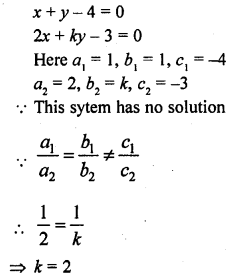Question 2.
Write the value of k for which the system of equations 2x – y = 5 6x + ky = 15 has infinitely many solutions.
Solution:Question 3.
Write the value of k for which the system of equations 3x – 2y = 0 and kx + 5y = 0 has infinitely many solutions.
Solution:
3x – 2y = 0
kx + 5y = 0
Here a1 = 3, b1 = -2, c1 = 0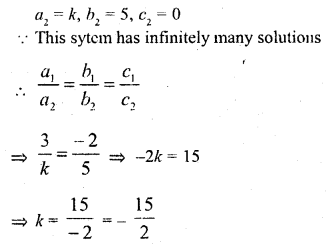Question 4.
Write the value of k for which the system of equations x + ky = 0, 2x – y = 0 has unique solution.
Solution:Question 5.
Write the set of values of a and b for which the following system of equations has infinitely many solutions.
2x + 3y = 7
2ax + (a + b) y = 28
Solution:Question 6.
For what value of ft, the following pair of linear equations has infinitely many soutions.
10x + 5y – (k – 5) = 0
20x + 10y – k = 0
Solution: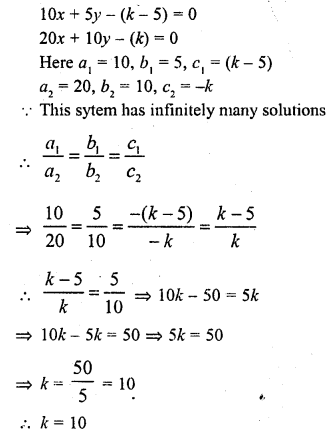Question 7.
Write the number of solutions of the following pair of linear equations :
x + 2y – 8 = 0
2x + 4y = 16 (C.B.S.E. 2009)
Solution:
x + 2y – 8 = 0 => x + 2y = 8 ….(i)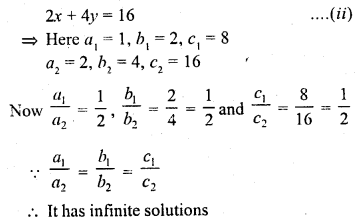Question 8.
Write the number of solutions of the following pair of linear equations :
x + 3y – 4 = 0
2x + 6y = 7
Solution: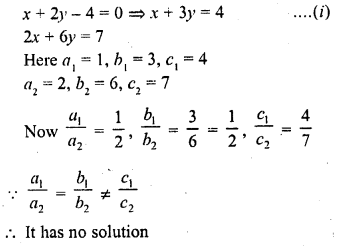Hope given RD Sharma Class 10 Solutions Chapter 3 Pair of Linear Equations in Two Variables VSAQS are helpful to complete your math homework.

If you have any doubts, please comment below. Learn Insta try to provide online math tutoring for you.